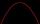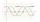# Examples of equations for 8th grade (13y)

#### Number of problems found: 863

• Simple equation 6Solve equation with one variable: X/2+X/3+X/4=X+4
• Equation with xSolve the following equation: 2x- (8x + 1) - (x + 2) / 5 = 9
• Equation with fractionsSolve equation: ? It is equation with fractions.
• Simply equationSolve this equation for x: ?
• EquationSolve the equation: 1/2-2/8 = 1/10; Write the result as a decimal number.
• Reciprocal equationDetermine the root of the equation: 9/x-7/x=1
• Equation with absSolve this equation with absolute value member: ?
• Diofant equationIn the set of integers (Z) solve the equation: ? Write result with integer parameter ? (parameter t = ...-2,-1,0,1,2,3... if equation has infinitely many solutions)
• Equation?Solve pure quadratic equation ?.
• Equation with mixed fractions2 3/5 of 1430+? = 1900. How to do this problem
• Eq with reciprocalSolve given equation with reciprocal member: a-6/a+10=4/8
• Exponential equationDetermine the value of having y in the expression (3^y): (4^-1)=36. Unknown y is a natural number greater than zero.
• EQ2Solve quadratic equation: ?
• SinusDetermine the smallest integer p for which the equation 4 sin x = p has no solution.
• SlopeWhat is the slope of the line defined by the equation -2x +3y = -1 ?
• Diofant 2Is equation ? solvable on the set of integers Z?
• Diofant equation250x + 120y = 5640
• RootThe root of the equation ? is (equal or greater or less than zero)? ?
• Equation - inverseSolve for x: 7: x = 14: 1000

Do you have an interesting mathematical word problem that you can't solve it? Submit a math problem, and we can try to solve it.

We will send a solution to your e-mail address. Solved examples are also published here. Please enter the e-mail correctly and check whether you don't have a full mailbox.

Please do not submit problems from current active competitions such as Mathematical Olympiad, correspondence seminars etc...

Do you have a linear equation or system of equations and looking for its solution? Or do you have quadratic equation?